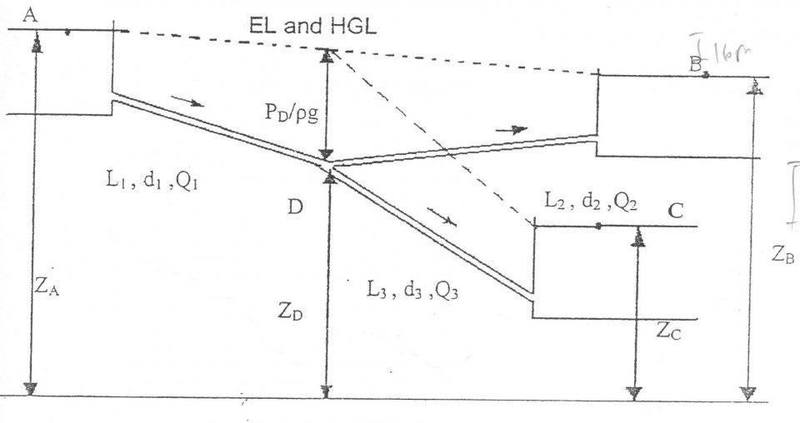# Flow in branch pipe 2

## Homework Statement

(post #14) , i was told that water can flow in one direction only in a netwrok of pipes .
However , in the notes uploaded here , the author stated that when P is below surface of B , then water must be out of B and Q1 + Q2 = Q3 ?## The Attempt at a Solution

IMO , it should be Q2 = Q1 + Q3 , since i was told that water can only flow in 1 direction , am i right ? [/B]

#### Attachments

Chestermiller
Mentor
I would not have set this problem up the way that they have set it up. I would have written the following 3 equations:

$$\frac{p_A}{\rho g}+z_A=\frac{p_D}{\rho g}+z_D+\frac{fL_1v_1^2}{gd_1}$$
$$\frac{p_D}{\rho g}+z_D=\frac{p_B}{\rho g}+z_B+\frac{fL_1v_2^2}{gd_1}$$
$$\frac{p_D}{\rho g}+z_D=\frac{p_C}{\rho g}+z_C+\frac{fL_1v_3^2}{gd_1}$$
So,
$$z_A=\frac{p_D}{\rho g}+z_D+\frac{fL_1v_1^2}{gd_1}\tag{1}$$
$$\frac{p_D}{\rho g}+z_D=z_B+\frac{fL_1v_2^2}{gd_1}\tag{2}$$
$$\frac{p_D}{\rho g}+z_D=z_C+\frac{fL_1v_3^2}{gd_1}\tag{3}$$

What would Eqn. 2 have to look like if the flow were from B to D, rather than from D to B?

•foo9008
I would not have set this problem up the way that they have set it up. I would have written the following 3 equations:

$$\frac{p_A}{\rho g}+z_A=\frac{p_D}{\rho g}+z_D+\frac{fL_1v_1^2}{gd_1}$$
$$\frac{p_D}{\rho g}+z_D=\frac{p_B}{\rho g}+z_B+\frac{fL_1v_2^2}{gd_1}$$
$$\frac{p_D}{\rho g}+z_D=\frac{p_C}{\rho g}+z_C+\frac{fL_1v_3^2}{gd_1}$$
So,
$$z_A=\frac{p_D}{\rho g}+z_D+\frac{fL_1v_1^2}{gd_1}\tag{1}$$
$$\frac{p_D}{\rho g}+z_D=z_B+\frac{fL_1v_2^2}{gd_1}\tag{2}$$
$$\frac{p_D}{\rho g}+z_D=z_C+\frac{fL_1v_3^2}{gd_1}\tag{3}$$

What would Eqn. 2 have to look like if the flow were from B to D, rather than from D to B?
if the flow were from B to D ,
PB / ρg + zB = PD / ρg + zD +f(L_2)[(v_2)^2] / 2g(D_2) ,
as PB = 0 ,so ,
zB = PD / ρg + zD +f(L_2)[(v_2)^2] / 2g(D_2) , am i right ?
am i right ?

Last edited:
is the author wrong ? how could Q1 + Q2 = Q3 ? how could the water flow in different direction ?
in the previous thread , i was told that in order for water from Res. B to flow to the split at D, it must flow against the pressure in the line created by the flow from Res. A , so the water can only flow out from A , and into B and C..

Chestermiller
Mentor
if the flow were from B to D ,
PB / ρg + zB = PD / ρg + zD +f(L_2)[(v_2)^2] / 2g(D_2) ,
as PB = 0 ,so ,
zB = PD / ρg + zD +f(L_2)[(v_2)^2] / 2g(D_2) , am i right ?
am i right ?
Yes. Now, see if there is a possible solution for this case.

Yes. Now, see if there is a possible solution for this case.
what do you mean ?

Chestermiller
Mentor
what do you mean ?
This would be applicable to the case where Q1+Q2 = Q3. Do the equations have a solution for this case?

This would be applicable to the case where Q1+Q2 = Q3. Do the equations have a solution for this case?
IMO , that is not feasible , since i was told that water can only flow in 1 direction

Chestermiller
Mentor
IMO , that is not feasible , since i was told that water can only flow in 1 direction
If that's the case, then when you solve the equations, you will not get a real solution. Why don't you try solving it and see what you get. Who told you that silly thing anyway?

•foo9008
If that's the case, then when you solve the equations, you will not get a real solution. Why don't you try solving it and see what you get. Who told you that silly thing anyway?
so , the statement of water can only flowing in 1 direction is incorrect ?

anyone can clarify ?

This would be applicable to the case where Q1+Q2 = Q3. Do the equations have a solution for this case?
how to know that if the equations have solutions ?

Chestermiller
Mentor
anyone can clarify ?
What direction would it be if D1 were equal to zero?

Chestermiller
Mentor
how to know that if the equations have solutions ?
Solve them and see if the solution is real or complex.